# Part A When titrated with a 0.1198 M solution of sodium hydroxide, a 58.00 mL solution...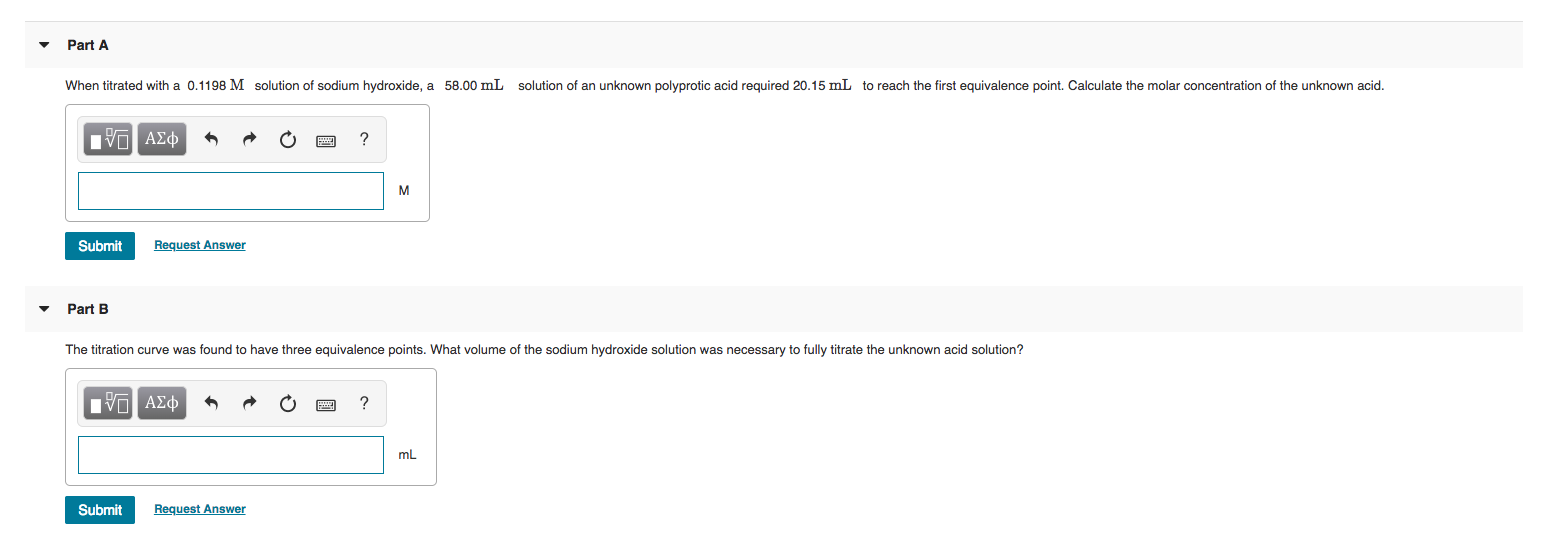Part A When titrated with a 0.1198 M solution of sodium hydroxide, a 58.00 mL solution of an unknown polyprotic acid required 20.15 mL to reach the first equivalence point. Calculate the molar concentration of the unknown acid. O A¢ * R O ? Submit Request Answer Part B The titration curve was found to have three equivalence points. What volume of the sodium hydroxide solution was necessary to fully titrate the unknown acid solution? IVO ADD A O O ? Submit Request Answer

Part A : molar concentration of unknown acid = 0.04162 M

Part B : volume of NaOH required = 60.45 mL

Explanation

Part A :

concentration NaOH = 0.1198 M

volume NaOH = 20.15 mL

moles NaOH = (concentration NaOH) * (volume NaOH)

moles NaOH = (0.1198 M) * (20.15 mL)

moles NaOH = 2.414 mmol

moles acid present = 2.414 mmol

concentration of acid = (moles acid present) / (volume of acid)

concentration of acid = (2.414 mmol) / (58.00 mL)

concentration of acid = 0.04162 M

#### Earn Coin

Coins can be redeemed for fabulous gifts.

Similar Homework Help Questions
• ### Part A When titrated with a 0.1038 M solution of sodium hydroxide, a 52.00 mL solution...Part A When titrated with a 0.1038 M solution of sodium hydroxide, a 52.00 mL solution of an unknown polyprotic acid required 22.86 mL to reach the first equivalence point. Calculate the molar concentration of the unknown acid. VALDO ? Submit Request Answer

• ### A sodium hydroxide solution of unknown concentration is titrated against 0.8765 g KHPh (Potassium acid phalate, a monoprotic acid, MW 204.3 g/mol)

1.A sodium hydroxide solution of unknown concentration is titrated against 0.8765 g KHPh (Potassium acid phalate, a monoprotic acid, MW 204.3 g/mol). 48.6 mL of the sodium hydroxide solution is required to reach the endpoint of the titration. What is the concentration of the unknown sodium hydroxide solution. 2.This sodium hydroxide solution is then used to titrate an unknown nitric acid sample. 25.0 mL of the nitric acid solution is titrated. 32.8 mL of the sodium hydroxide solution is required...

• ### If 25 mL of nitric acid is titrated with 0.12 M sodium hydroxide and it took...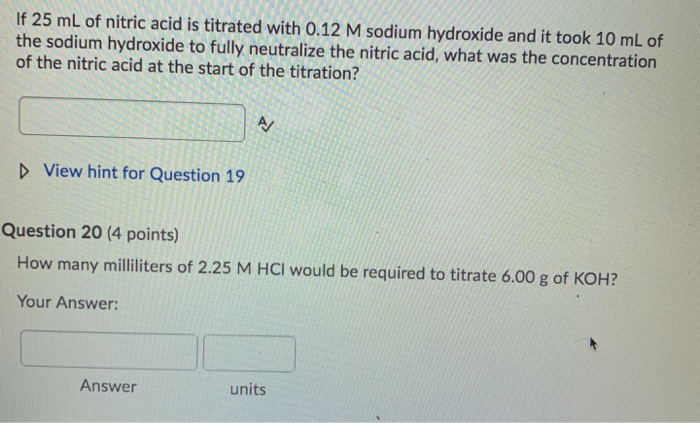If 25 mL of nitric acid is titrated with 0.12 M sodium hydroxide and it took 10 mL of the sodium hydroxide to fully neutralize the nitric acid, what was the concentration of the nitric acid at the start of the titration? D View hint for Question 19 Question 20 (4 points) How many milliliters of 2.25 M HCl would be required to titrate 6.00 g of KOH? Your Answer: Answer units

• ### 2. Calculate the mass of solid sodium hydroxide needed to prepare 300 mL of 0.1 M...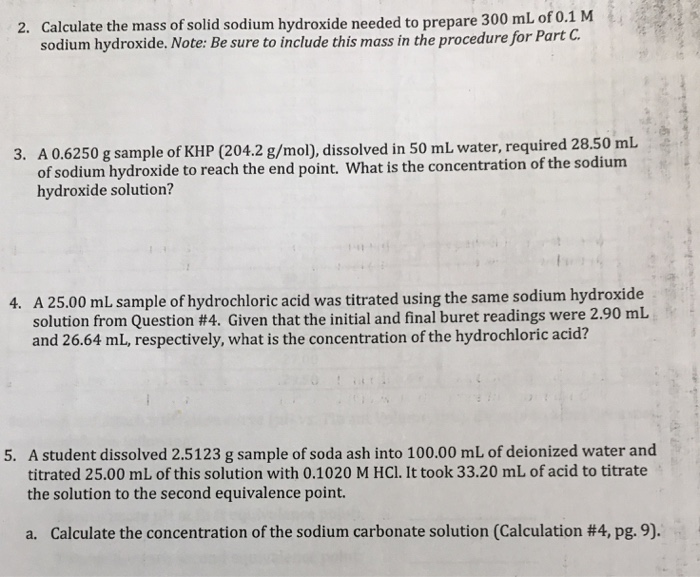2. Calculate the mass of solid sodium hydroxide needed to prepare 300 mL of 0.1 M sodium hydroxide. Note: Be sure to include this mass in the procedure for Part C. 3. A 0.6250 g sample of KHP (204.2 g/mol), dissolved in 50 mL water, required 28.50 mL of sodium hydroxide to reach the end point. What is the concentration of the sodium hydroxide solution? 4. A 25.00 mL sample of hydrochloric acid was titrated using the same sodium hydroxide...

• ### In a titration, 25 mL of 0.10 M weak diprotic acid solution was titrated by 0.10 M sodium hydroxide, NaOH, and produ...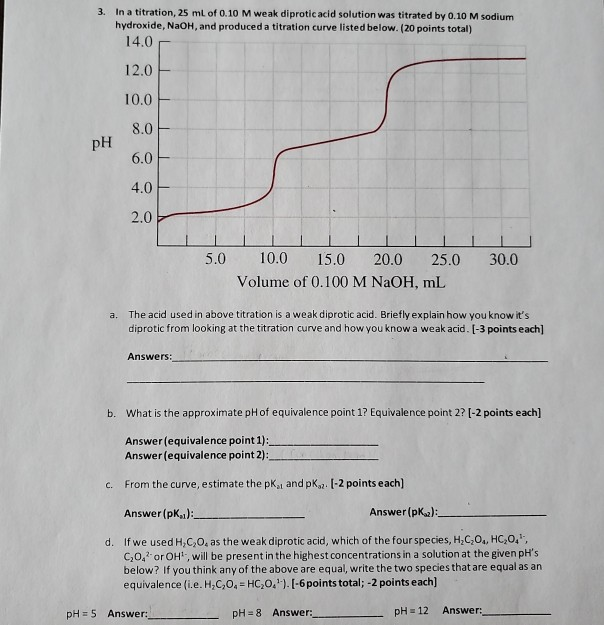In a titration, 25 mL of 0.10 M weak diprotic acid solution was titrated by 0.10 M sodium hydroxide, NaOH, and produced a titration curve listed below. (20 points total) 14,0 3. 12.0 10.0 8.0 pH 6.0 4.0 2.0 10.0 5.0 20.0 30.0 15.0 25.0 Volume of 0.100 M NaOH, mL The acid used in above titration is a weak diprotic acid. Briefly explain how you know it's diprotic from looking at the titration curve and how you know a...

• ### Q. #11. 25.00 mL of 0.100 M HNO, acid is titrated with 0.110 M sodium hydroxide...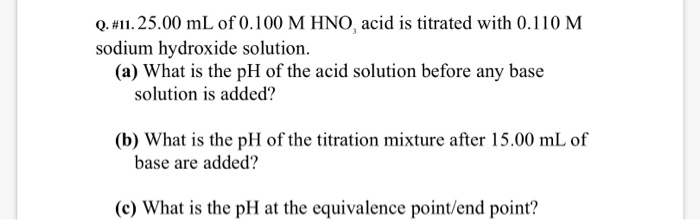Q. #11. 25.00 mL of 0.100 M HNO, acid is titrated with 0.110 M sodium hydroxide solution (a) What is the pH of the acid solution before any base solution is added? (b) What is the pH of the titration mixture after 15.00 mL of base are added? (c) What is the pH at the equivalence point/end point?

• ### When a 29,8 mL sample of a 0.322 M aqueous acetic acid solution is titrated with...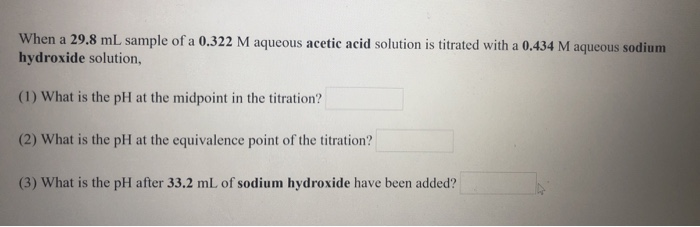When a 29,8 mL sample of a 0.322 M aqueous acetic acid solution is titrated with a 0,434 M aqueous sodium hydroxide solution, (1) What is the pH at the midpoint in the titration? (2) What is the pH at the equivalence point of the titration? (3) What is the pH after 33.2 mL of sodium hydroxide have been added?

• ### When a 23.8 mL sample of a 0.443 M aqueous hydrofluorie acid solution is titrated with...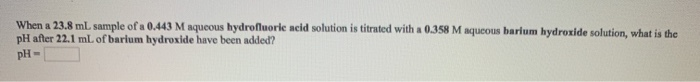When a 23.8 mL sample of a 0.443 M aqueous hydrofluorie acid solution is titrated with a 0.358 M aqueous barium hydroxide solution, what is the pH after 22.1 mL of barium hydroxide have been added? pH- What is the pH at the equivalence point in the titration of a 19.7 mL sample of a 0.376 M aqueous nitrous acid solution with a 0.447 M aqueous sodium hydroxide solution? pH- When a 20.1 mL sample of a 0.417 M aqueous...

• ### Questions 1. Calculate the molarity of a sodium hydroxide (NaOH) solution that is titrated with 0.6887...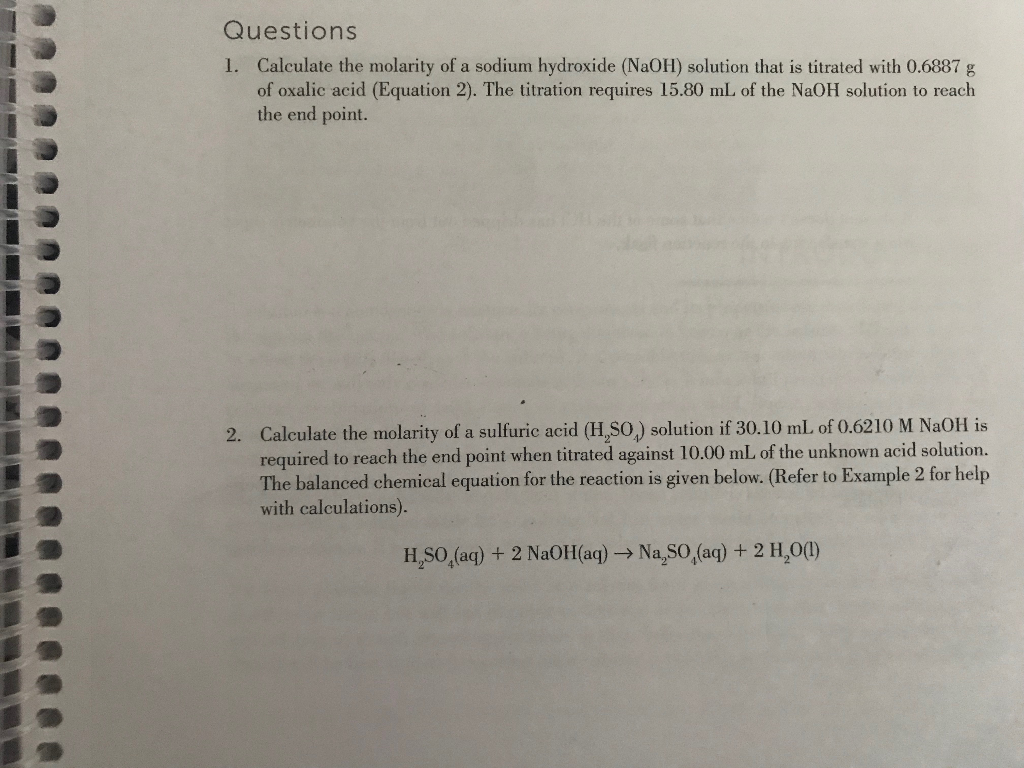Questions 1. Calculate the molarity of a sodium hydroxide (NaOH) solution that is titrated with 0.6887 g of oxalic acid (Equation 2). The titration requires 15.80 mL of the NaOH solution to reach the end point. Calculate the molarity of a sulfuric acid (H,SO) solution if 30.10 mL of 0.62 10 M NaOH is required to reach the end point when titrated against 10.00 mL of the unknown acid solution. The balanced chemical equation for the reaction is given below....

• ### When a 23.2 mL sample of a 0.345 M aqueous acetic acid solution is titrated with...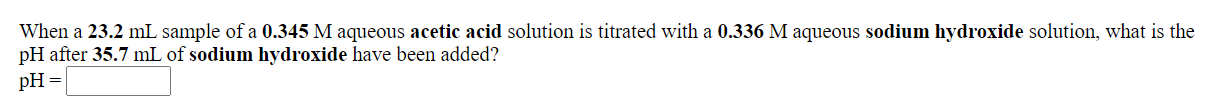When a 23.2 mL sample of a 0.345 M aqueous acetic acid solution is titrated with a 0.336 M aqueous sodium hydroxide solution, what is the pH after 35.7 mL of sodium hydroxide have been added? pH = What is the pH at the equivalence point in the titration of a 27.6 mL sample of a 0.423 M aqueous hydrocyanic acid solution with a 0.431 M aqueous potassium hydroxide solution? pH=